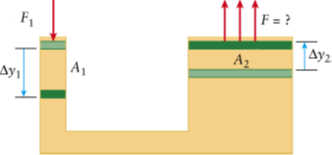# Problem: The hydraulic lift (the figure below) has a small piston with the surface  A1= 0.039 m2 and a large piston with the surface A2 = 5.0 m2. F1 = 21 N. What force will the large piston provide if the small piston in a hydraulic lift is moved down as shown in the figure? (Express your answer to two significant figures.)

🤓 Based on our data, we think this question is relevant for Professor Barkan's class at UIC.

###### Problem Details

The hydraulic lift (the figure below) has a small piston with the surface  A1= 0.039 m2 and a large piston with the surface A= 5.0 m2. F= 21 N. What force will the large piston provide if the small piston in a hydraulic lift is moved down as shown in the figure? (Express your answer to two significant figures.)